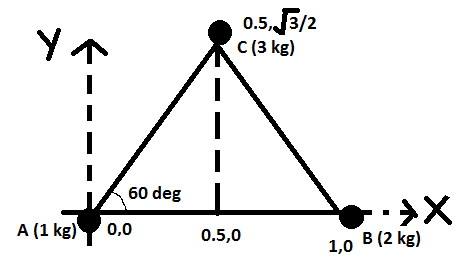# three particle of mass 1 kg,2kg and 3kg are placed at the cornners A, B and C respictavely of an equilateral tringle ABC of edgs 1 m . find the distance of their center of mass from A.

10 years ago

Hi jitendra,

let ABC be the reqd. triangle.

Let A lie at the origin. Therefore, B will automatically lie at (1,0).

mid point of AB hall lie at (0.5,0). Angle A= 60 deg. (equilateral traingle)

√3/2= CD/1

CD=√3/2

Therefore coordinates of C become (0.5, √3/2)now, COM can be easily calculated.

X= 1(0)+2(1) + 3(0.5)/1+2+3 = 0.583

Y= 1(0) + 2(0)+ 3(√3/2)/6 = √3/4=0.433

distance of com from A= Distance of com from origin = (0.583 i + 0.433 j)

if you wan tto further simplify,

it can easily be calculated as √(0.583)2+(0.433)2

= √0.339+0.187

= 0.725 metres from A